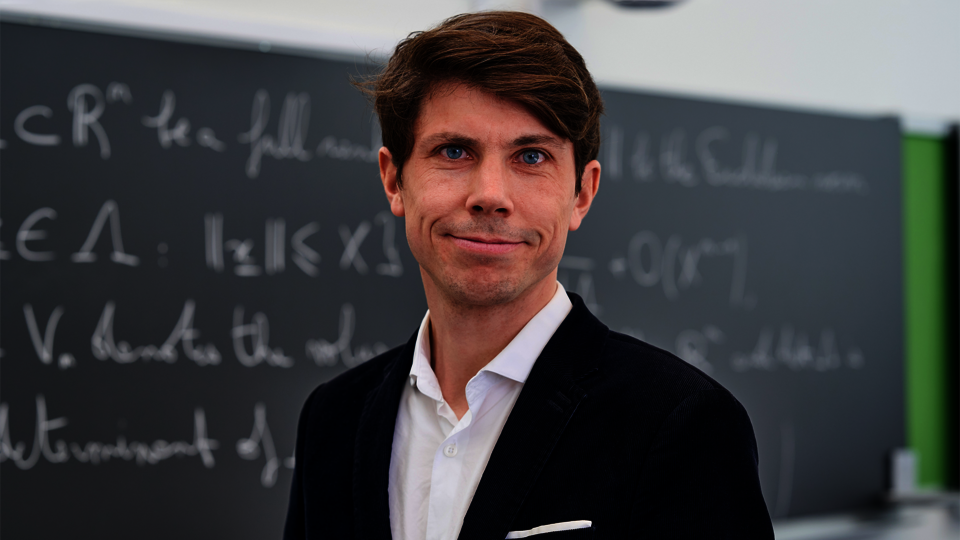/ Jana Winkler

## As close as possible to solving Hilbert's tenth problemDavid Hilbert posed the question whether or not it would be possible to develop an algorithm that was able to find out whether polynomial equations in several variables with integer coefficients also have integer solutions. This question became known as Hilbert's tenth problem. Although it generally cannot be solved, a team of mathematicians came as close as possible.

Professor Pierre Le Boudec of the University of Basel, Professor Tim Browning of the Institute of Science and Technology Austria, and Professor Will Sawin of Columbia University have presented a new theorem that provides the key to solving Hilbert's tenth problem for almost all polynomials whose number of variables are greater than their degree. When they first presented their results in Paris in 2019, it was “immediately noticeable from the audience's reaction that we had contributed something really significant“ Prof. Le Boudec remembers. Their research results represent a groundbreaking advance in number theory and were now published in the prestigious journal Annals of Mathematics.

During their time on the project, the mathematicians around Pierre Le Boudec were not concerned with finding integer solutions for all polynomials. They decided to adopt a statistical perspective on this problem: Provided that a given polynomial equation has the so-called local solutions in all completions of the field of rational numbers, it can be predicted with high probability that it also has an integer solution. These completions were classified by Alexander Ostrowski, a mathematician
working in Basel. It is known that checking the existence of these local solutions can be done algorithmically. Prof. Le Boudec and his co-authors were able to prove a conjecture of Poonen and Voloch, which had been open for almost 20 years, except for cubic surfaces.

So the algorithm Hilbert longed for exists, at least statistically, in most cases. Thus, the researchers may have come as close as possible to solving Hilbert's tenth problem.

The results of Pierre Le Boudec, Tim Browning and Will Sawin have contributed significantly to the progress of basic research in the field of number theory and have extended the structural understanding of polynomial equations.